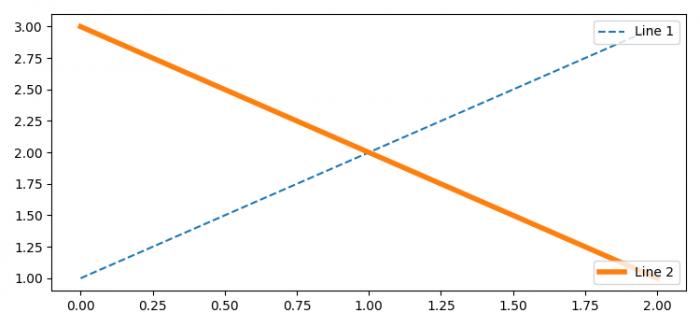# Drawing multiple legends on the same axes in Matplotlib

MatplotlibPythonData Visualization

To draw multiple legends on the same axes in Matplotlib, we can take the following steps −

• Set the figure size and adjust the padding between and around the subplots
• Plot lines using two lists with different labels, linewidth and linestyle.
• Place the first legend at the upper-right location.
• Add artist, i.e., first legend on the current axis.
• Place the second legend on the current axis at the lower-right location.
• To display the figure, use show() method.

## Example

from matplotlib import pyplot as plt

plt.rcParams["figure.figsize"] = [7.50, 3.50]
plt.rcParams["figure.autolayout"] = True

line1, = plt.plot([1, 2, 3], label="Line 1", linestyle='--')
line2, = plt.plot([3, 2, 1], label="Line 2", linewidth=4)

first_legend = plt.legend(handles=[line1], loc='upper right')

plt.show()